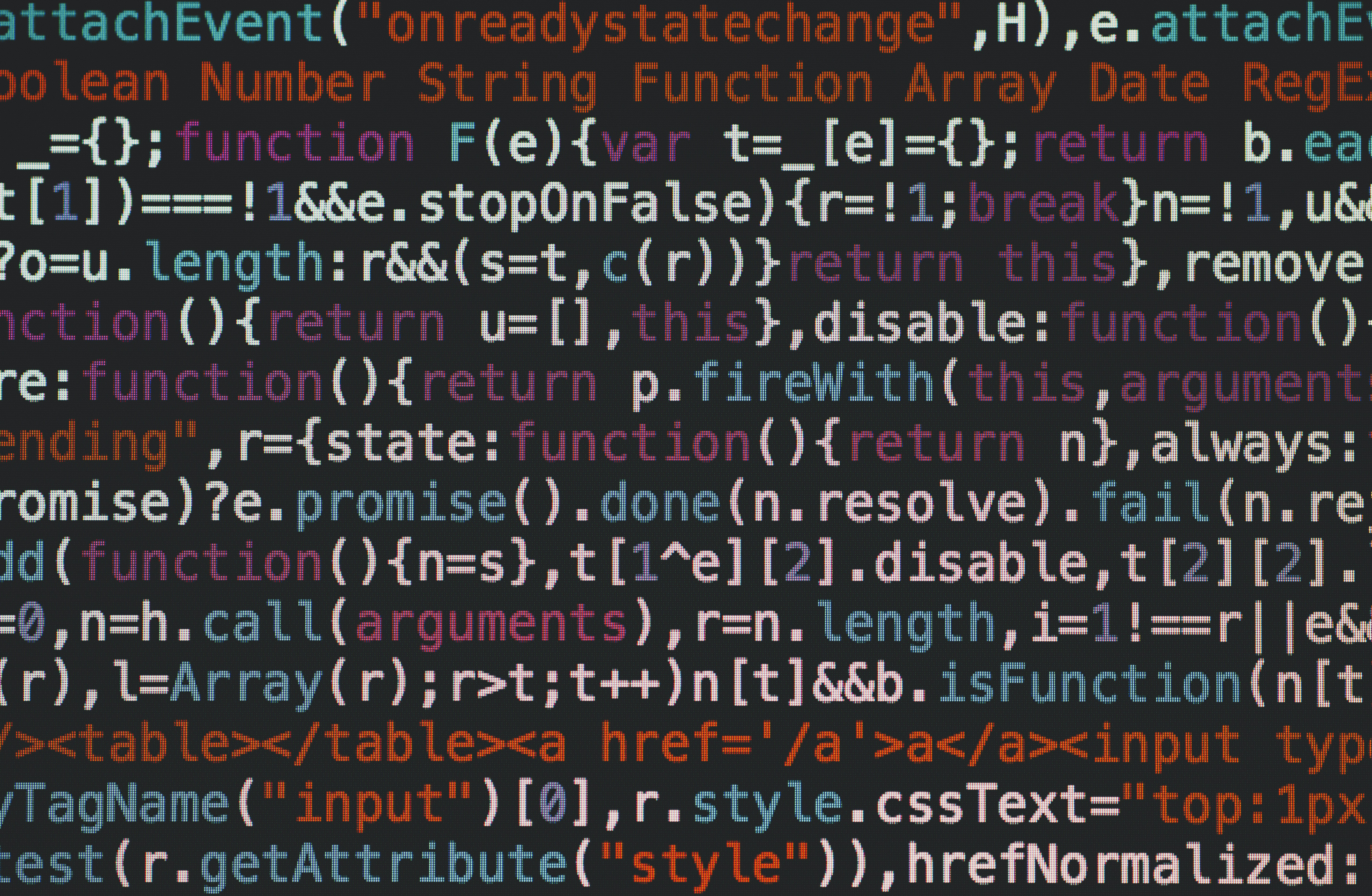July 15, 2018

# Programming Challenge: N Digits

Today's challenge: given a number of digits, print the sum of all numbers that can be expressed using all such digits.Today's challenge: given a number of digits, print the sum of all numbers that can be expressed using all such digits.

That's simple enough, right? So, give us the sum for the input `54321`.

Here's a quick example: for the digits 1 and 2, the two numbers that can be expressed are 12 and 21, which gives us a sum of 33.

``````Mzk5OTk2MAo=